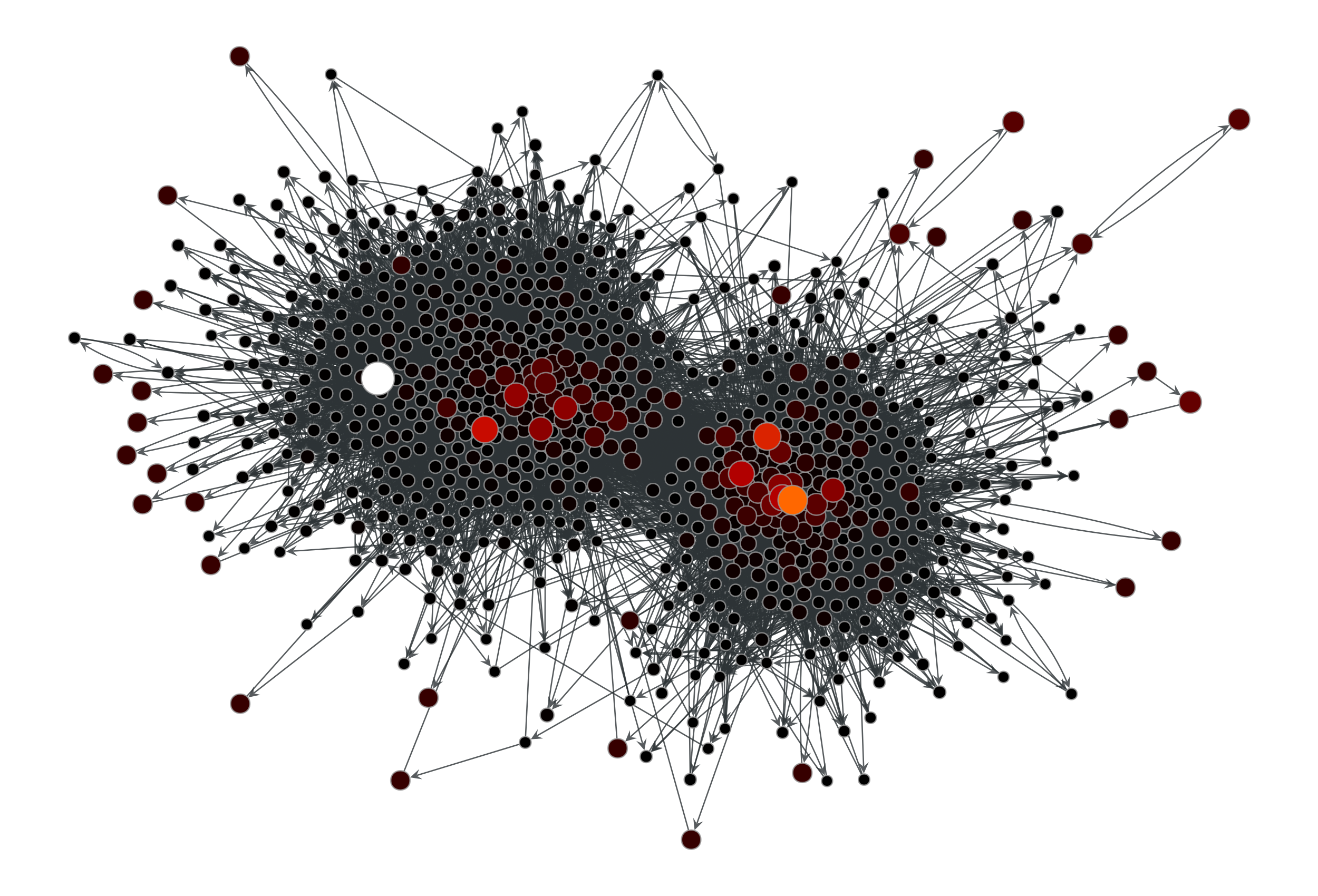# graph_tool.centrality.pagerank#

graph_tool.centrality.pagerank(g, damping=0.85, pers=None, weight=None, prop=None, epsilon=1e-06, max_iter=None, ret_iter=False)[source]#

Calculate the PageRank of each vertex.

Parameters:
gGraph

Graph to be used.

dampingfloat, optional (default: 0.85)

Damping factor.

persVertexPropertyMap, optional (default: None)

Personalization vector. If omitted, a constant value of $$1/N$$ will be used.

weightEdgePropertyMap, optional (default: None)

Edge weights. If omitted, a constant value of 1 will be used.

propVertexPropertyMap, optional (default: None)

Vertex property map to store the PageRank values. If supplied, it will be used uninitialized.

epsilonfloat, optional (default: 1e-6)

Convergence condition. The iteration will stop if the total delta of all vertices are below this value.

max_iterint, optional (default: None)

If supplied, this will limit the total number of iterations.

ret_iterbool, optional (default: False)

If true, the total number of iterations is also returned.

Returns:
pagerankVertexPropertyMap

A vertex property map containing the PageRank values.

betweenness

betweenness centrality

eigentrust

eigentrust centrality

eigenvector

eigenvector centrality

hits

authority and hub centralities

trust_transitivity

pervasive trust transitivity

Notes

The value of PageRank [pagerank-wikipedia] of vertex v, $$PR(v)$$, is given iteratively by the relation:

$PR(v) = \frac{1-d}{N} + d \sum_{u \in \Gamma^{-}(v)} \frac{PR (u)}{d^{+}(u)}$

where $$\Gamma^{-}(v)$$ are the in-neighbors of v, $$d^{+}(u)$$ is the out-degree of u, and d is a damping factor.

If a personalization property $$p(v)$$ is given, the definition becomes:

$PR(v) = (1-d)p(v) + d \sum_{u \in \Gamma^{-}(v)} \frac{PR (u)}{d^{+}(u)}$

If edge weights are also given, the equation is then generalized to:

$PR(v) = (1-d)p(v) + d \sum_{u \in \Gamma^{-}(v)} \frac{PR (u) w_{u\to v}}{d^{+}(u)}$

where $$d^{+}(u)=\sum_{y}A_{u,y}w_{u\to y}$$ is redefined to be the sum of the weights of the out-going edges from u.

If a node has out-degree zero, it is assumed to connect to every other node with a weight proportional to $$p(v)$$ or a constant if no personalization is given.

The implemented algorithm progressively iterates the above equations, until it no longer changes, according to the parameter epsilon. It has a topology-dependent running time.

If enabled during compilation, this algorithm runs in parallel.

References

[lawrence-pagerank-1998]

P. Lawrence, B. Sergey, M. Rajeev, W. Terry, “The pagerank citation ranking: Bringing order to the web”, Technical report, Stanford University, 1998

[Langville-survey-2005]

A. N. Langville, C. D. Meyer, “A Survey of Eigenvector Methods for Web Information Retrieval”, SIAM Review, vol. 47, no. 1, pp. 135-161, 2005, DOI: 10.1137/S0036144503424786 [sci-hub, @tor]

L. A. Adamic and N. Glance, “The political blogosphere and the 2004 US Election”, in Proceedings of the WWW-2005 Workshop on the Weblogging Ecosystem (2005). DOI: 10.1145/1134271.1134277 [sci-hub, @tor]

Examples

>>> g = gt.collection.data["polblogs"]
>>> g = gt.GraphView(g, vfilt=gt.label_largest_component(g))
>>> pr = gt.pagerank(g)
>>> gt.graph_draw(g, pos=g.vp["pos"], vertex_fill_color=pr,
...               vertex_size=gt.prop_to_size(pr, mi=5, ma=15),
...               vorder=pr, vcmap=matplotlib.cm.gist_heat,
...               output="polblogs_pr.pdf")
<...>


Now with a personalization vector, and edge weights:

>>> d = g.degree_property_map("total")
>>> periphery = d.a <= 2
>>> p = g.new_vertex_property("double")
>>> p.a[periphery] = 100
>>> pr = gt.pagerank(g, pers=p)
>>> gt.graph_draw(g, pos=g.vp["pos"], vertex_fill_color=pr,
...               vertex_size=gt.prop_to_size(pr, mi=5, ma=15),
...               vorder=pr, vcmap=matplotlib.cm.gist_heat,
...               output="polblogs_pr_pers.pdf")
<...>Personalized PageRank values of the a political blogs network of [adamic-polblogs], where vertices with very low degree are given artificially high scores.#